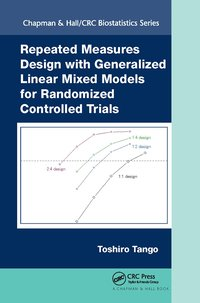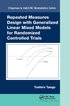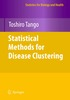Fler böcker inom
Format
Inbunden (Hardback)
Språk
Engelska
Antal sidor
360
Utgivningsdatum
2017-02-16
Förlag
Productivity Press
Antal komponenter
1
ISBN
9781498747899

### Du kanske gillar# Repeated Measures Design with Generalized Linear Mixed Models for Randomized Controlled Trials

Inbunden Engelska, 2017-02-16
949
• Skickas inom 7-10 vardagar.
• Gratis frakt inom Sverige över 159 kr för privatpersoner.
Finns även som
489
489
• Skickas inom 10-15 vardagar
599
Repeated Measures Design with Generalized Linear Mixed Models for Randomized Controlled Trials is the first book focused on the application of generalized linear mixed models and its related models in the statistical design and analysis of repeated measures from randomized controlled trials. The author introduces a new repeated measures design called S:T design combined with mixed models as a practical and useful framework of parallel group RCT design because of easy handling of missing data and sample size reduction. The book emphasizes practical, rather than theoretical, aspects of statistical analyses and the interpretation of results. It includes chapters in which the author describes some old-fashioned analysis designs that have been in the literature and compares the results with those obtained from the corresponding mixed models. The book will be of interest to biostatisticians, researchers, and graduate students in the medical and health sciences who are involved in clinical trials. Author Website: Data sets and programs used in the book are available at http://www.medstat.jp/downloadrepeatedcrc.html

## Passar bra ihop

1.2. +
3.De som köpt den här boken har ofta också köpt Statistical Methods for Disease Clustering av Toshiro Tango (inbunden).

## Kundrecensioner

Har du läst boken? Sätt ditt betyg »

## Fler böcker av Toshiro Tango

• ### Statistical Methods for Disease Clustering

#### Toshiro Tango

This book is intended to provide a text on statistical methods for detecting clus ters and/or clustering of health events that is of interest to ?nal year undergraduate and graduate level statistics, biostatistics, epidemiology, and geography stud...

## Recensioner i media

"The main focus of this book is to introduce the generalized linear mixed-effects models with S:T repeated measures design, which provide a flexible and powerful tool to deal with longitudinal data with heterogeneity or variability among subject-specific responses and missing data. This book illustrates theoretical methodologies with a focus on the practicality with a wealth of real-life examples making it easy to understand the topics. It is well organized and contains SAS codes and outputs as useful references. In summary, this is an excellent book with a very good selection of examples. It is clearly written and is enjoyable to read." ~Misoo C. Ellison, Merck & Co., Inc., Kenilworth, NJ

## Övrig information

Toshiro Tango is the Director of Center for Medical Statistics, Tokyo. His research interests include various aspects of biostatistics including design and analysis of clinical trials and spatial epidemiology. He has served as associate editor for several journals including Biometrics and Statistics in Medicine, and is the author of Statistical Methods for Disease Clustering.

## Innehållsförteckning

Introduction Repeated measures design Generalized linear mixed models Model for the treatment effect at each scheduled visit Model for the average treatment effect Model for the treatment by linear time interaction Superiority and non-inferiority Naive analysis of animal experiment data Introduction Analysis plan I Analysis plan II each time point Analysis plan III - analysis of covariance at the last time point Discussion Analysis of variance models Introduction Analysis of variance model Change from baseline Split-plot design Selecting a good _t covariance structure using SAS Heterogeneous covariance ANCOVA-type models From ANOVA models to mixed-effects repeated measures models Introduction Shift to mixed-effects repeated measures models ANCOVA-type mixed-effects models Unbiased estimator for treatment effects Illustration of the mixed-effects models Introduction The Growth data Linear regression model Random intercept model Random intercept plus slope model Analysis using The Rat data Random intercept Random intercept plus slope Random intercept plus slope model with slopes varying over time Likelihood-based ignorable analysis for missing data Introduction Handling of missing data Likelihood-based ignorable analysis Sensitivity analysis The Growth The Rat data MMRM vs. LOCF Mixed-effects normal linear regression models Example: The Beat the Blues data with 1:4 design Checking missing data mechanism via a graphical procedure Data format for analysis using SAS Models for the treatment effect at each scheduled visit Model I: Random intercept model Model II: Random intercept plus slope model Model III: Random intercept plus slope model with slopes varying over time Analysis using SAS Models for the average treatment effect Model IV: Random intercept model Model V: Random intercept plus slope model Analysis using SAS Heteroscedastic models Models for the treatment by linear time interaction Model VI: Random intercept model Model VII: Random intercept plus slope model Analysis using SAS Checking the goodness-of-_t of linearity ANCOVA-type models adjusting for baseline measurement Model VIII: Random intercept model for the treatment effect at each visit Model IX: Random intercept model for the average treatment effect Analysis using SAS Sample size Sample size for the average treatment effect Sample size assuming no missing data Sample size allowing for missing data Sample size for the treatment by linear time interaction Discussion Mixed-effects logistic regression models The Respiratory data with 1:4 design Odds ratio Logistic regression models Models for the treatment effect at each scheduled visit Model I: Random intercept model Model II: Random intercept plus slope model Analysis using SAS Models for the average treatment effect Model IV: Random intercept model Model V: Random intercept plus slope model Analysis using SAS Models for the treatment by linear time interaction Model VI: Random intercept model Model VII: Random intercept plus slope model Analysis using SAS Checking the goodness-of-_t of linearity ANCOVA-type models adjusting for baseline measurement Model VIII: Random intercept model for the treatment effect at each visit Model IX: Random intercept model for the average treatment effect Analysis using SAS The daily symptom data with 7:7 design Models for the average treatment effect Analysis using SAS Sample size Sample size for the average treatment effect Sample size for the treatment by linear time interaction Mixed-effects Poisson regression models The Epilepsy data with 1:4 design Rate Ratio Poisson regression models Models for the treatment effect at each scheduled visit Model I: Random intercept